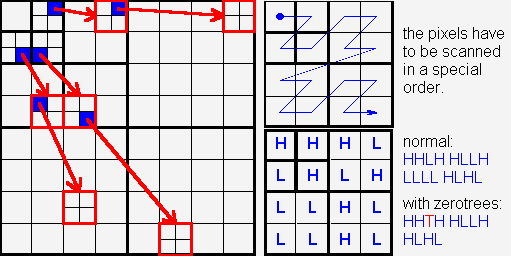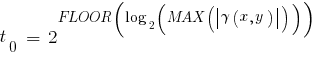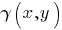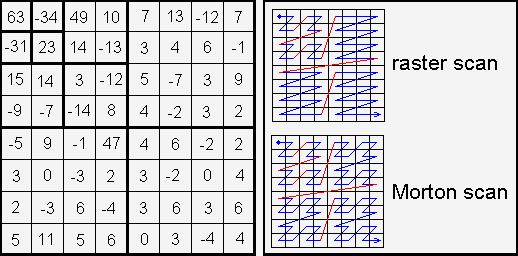# EZW Encoding

## 1. Introduction

In this text I will try to explain the implementation of an Embedded Zerotree Wavelet encoder or EZW encoder, which was presented in a paper by J. Shapiro [Sha93]. The reason for this is that I have never come across a good explanation of this technique, yet many researchers claim that they have implemented it. Of course there is Shapiro’s original paper but when reading his paper carefully many details are not immediately clear or even missing. Since I think that the approach of EZW encoding is a fruitful one (see for instance [Cre97]) I have decided to present the details here. This might save others some work in the future.

This text expects some understanding of wavelet transforms.

## 2. EZW encoding

When searching through wavelet literature for image compression schemes it is almost impossible not to note Shapiro’s Embedded Zerotree Wavelet encoder or EZW encoder for short [Sha93]. An EZW encoder is an encoder specially designed to use with wavelet transforms, which explains why it has the word wavelet in its name. The EZW encoder was originally designed to operate on images (2D-signals) but it can also be used on other dimensional signals.

The EZW encoder is based on progressive encoding to compress an image into a bit stream with increasing accuracy. This means that when more bits are added to the stream, the decoded image will contain more detail, a property similar to JPEG encoded images. It is also similar to the representation of a number like pi. Every digit we add increases the accuracy of the number, but we can stop at any accuracy we like. Progressive encoding is also known as embedded encoding, which explains the E in EZW.

This leaves us with the Z. This letter is a bit more complicated to explain, but I will give it a try in the next paragraph.

Coding an image using the EZW scheme, together with some optimizations results in a remarkably effective image compressor with the property that the compressed data stream can have any bit rate desired. Any bit rate is only possible if there is information loss somewhere so that the compressor is lossy. However, lossless compression is also possible with an EZW encoder, but of course with less spectacular results.

## 3. The zerotree

The EZW encoder is based on two important observations:

1. Natural images in general have a low pass spectrum. When an image is wavelet transformed the energy in the subbands decreases as the scale decreases (low scale means high resolution), so the wavelet coefficients will, on average, be smaller in the higher subbands than in the lower subbands. This shows that progressive encoding is a very natural choice for compressing wavelet transformed images, since the higher subbands only add detail;
2. Large wavelet coefficients are more important than small wavelet coefficients.

These two observations are exploited by encoding the wavelet coefficients in decreasing order, in several passes. For every pass a threshold is chosen against which all the wavelet coefficients are measured. If a wavelet coefficient is larger than the threshold it is encoded and removed from the image, if it is smaller it is left for the next pass. When all the wavelet coefficients have been visited the threshold is lowered and the image is scanned again to add more detail to the already encoded image. This process is repeated until all the wavelet coefficients have been encoded completely or another criterion has been satisfied (maximum bit rate for instance).

The trick is now to use the dependency between the wavelet coefficients across different scales to efficiently encode large parts of the image which are below the current threshold. It is here where the zerotree enters. So, let me now add some detail to the foregoing. (As most explanations, this explanation is a progressive one.)

A wavelet transform transforms a signal from the time domain to the joint time-scale domain. This means that the wavelet coefficients are two-dimensional. If we want to compress the transformed signal we have to code not only the coefficient values, but also their position in time. When the signal is an image then the position in time is better expressed as the position in space. After wavelet transforming an image we can represent it using trees because of the subsampling that is performed in the transform. A coefficient in a low subband can be thought of as having four descendants in the next higher subband (see figure 1). The four descendants each also have four descendants in the next higher subband and we see a quad-tree emerge: every root has four leafs.

We can now give a definition of the zerotree. A zerotree is a quad-tree of which all nodes are equal to or smaller than the root. The tree is coded with a single symbol and reconstructed by the decoder as a quad-tree filled with zeroes. To clutter this definition we have to add that the root has to be smaller than the threshold against which the wavelet coefficients are currently being measured.

The EZW encoder exploits the zerotree based on the observation that wavelet coefficients decrease with scale. It assumes that there will be a very high probability that all the coefficients in a quad tree will be smaller than a certain threshold if the root is smaller than this threshold. If this is the case then the whole tree can be coded with a single zerotree symbol. Now if the image is scanned in a predefined order, going from high scale to low, implicitly many positions are coded through the use of zerotree symbols. Of course the zerotree rule will be violated often, but as it turns out in practice, the probability is still very high in general. The price to pay is the addition of the zerotree symbol to our code alphabet.

## 4. How does it work?

Now that we have all the terms defined we can start compressing. Let’s begin with the encoding of the coefficients in decreasing order.

A very direct approach is to simply transmit the values of the coefficients in decreasing order, but this is not very efficient. This way a lot of bits are spend on the coefficient values and we do not use the fact that we know that the coefficients are in decreasing order. A better approach is to use a threshold and only signal to the decoder if the values are larger or smaller than the threshold. If we also transmit the threshold to the decoder, it can reconstruct already quite a lot. To arrive at a perfect reconstruction we repeat the process after lowering the threshold, until the threshold has become smaller than the smallest coefficient we wanted to transmit. We can make this process much more efficient by subtracting the threshold from the values that were larger than the threshold. This results in a bit stream with increasing accuracy and which can be perfectly reconstructed by the decoder. If we use a predetermined sequence of thresholds then we do not have to transmit them to the decoder and thus save us some bandwidth. If the predetermined sequence is a sequence of powers of two it is called bitplane coding since the thresholds in this case correspond to the bits in the binary representation of the coefficients. EZW encoding as described in [Sha93] uses this type of coefficient value encoding.

One important thing is however still missing: the transmission of the coefficient positions. Indeed, without this information the decoder will not be able to reconstruct the encoded signal (although it can perfectly reconstruct the transmitted bit stream). It is in the encoding of the positions where the efficient encoders are separated from the inefficient ones.

As mentioned before, EZW encoding uses a predefined scan order to encode the position of the wavelet coefficients (see figure 2). Through the use of zerotrees many positions are encoded implicitly. Several scan orders are possible (see figure 3), as long as the lower subbands are completely scanned before going on to the higher subbands. In [Sha93] a raster scan order is used, while in [Alg95] some other scan orders are mentioned. The scan order seems to be of some influence of the final compression result.Figure 2. The relations between wavelet coefficients in different subbands (left), how to scan them (upper right) and the result of using zerotree (lower right) symbols (T) in the coding process. An H means that the coefficient is higher than the threshold and an L means that it is below the threshold. The zerotree symbol (T) replaces the four L's in the lower left part and the L in the upper left part.

Now that we know how the EZW scheme codes coefficient values and positions we can go on to the algorithm.

## 5. The algorithm

The EZW output stream will have to start with some information to synchronize the decoder. The minimum information required by the decoder is the number of wavelet transform levels used and the initial threshold, if we assume that always the same wavelet transform will be used. Additionally we can send the image dimensions and the image mean. Sending the image mean is useful if we remove it from the image before coding. After imperfect reconstruction the decoder can then replace the imperfect mean by the original mean. This can increase the PSNR significantly.

The first step in the EZW coding algorithm is to determine the initial threshold. If we adopt bitplane coding then our initial threshold t0 will beHere MAX(.) means the maximum coefficient value in the image anddenotes the coefficient. With this threshold we enter the main coding loop (I will use a C-like language):

```threshold = initial_threshold;
do
{
dominant_pass(image);
subordinate_pass(image);
threshold = threshold/2;
}
while (threshold>minimum_threshold);```

We see that two passes are used to code the image. In the first pass, the dominant pass, the image is scanned and a symbol is outputted for every coefficient. If the coefficient is larger than the threshold a P (positive) is coded, if the coefficient is smaller than minus the threshold an N (negative) is coded. If the coefficient is the root of a zerotree then a T (zerotree) is coded and finally, if the coefficient is smaller than the threshold but it is not the root of a zerotree, then a Z (isolated zero) is coded. This happens when there is a coefficient larger than the threshold in the subtree. The effect of using the N and P codes is that when a coefficient is found to be larger than the threshold (in absolute value or magnitude) its two most significant bits are outputted (if we forget about sign extension).

Note that in order to determine if a coefficient is the root of a zerotree or an isolated zero, we will have to scan the whole quad-tree. Clearly this will take time. Also, to prevent outputting codes for coefficients in already identified zerotrees we will have to keep track of them. This means memory for book keeping.

Finally, all the coefficients that are in absolute value larger than the current threshold are extracted and placed without their sign on the subordinate list and their positions in the image are filled with zeroes. This will prevent them from being coded again.

The second pass, the subordinate pass, is the refinement pass. In [Sha93] this gives rise to some juggling with uncertainty intervals, but it boils down to outputting the next most significant bit of all the coefficients on the subordinate list. In [Sha93] this list is ordered (in such a way that the decoder can do the same) so that the largest coefficients are again transmitted first. Based on [Alg95] we have not implemented this sorting here since the gain seems to be very small but the costs very high.

The main loop ends when the threshold reaches a minimum value. For integer coefficients this minimum value equals zero and the divide by two can be replaced by a shift right operation. If we add another ending condition based on the number of outputted bits by the arithmetic coder then we can meet any target bit rate exactly without doing too much work.

We can summarize the above with the following code fragments, starting with the dominant pass.

```/*
* Dominant pass
*/
initialize_fifo();
while (fifo_not_empty)
{
get_coded_coefficient_from_fifo();
if coefficient was coded as P, N or Z then
{
code_next_scan_coefficient();
put_coded_coefficient_in_fifo();
if coefficient was coded as P or N then
{
set coefficient position to zero;
}
}
}```

Here we have used a FIFO to keep track of the identified zerotrees. If we want to enter this loop we will have to initialize the FIFO by “manually” adding the first quad-tree root coefficients to the FIFO. Depending on which level we start in the left of figure 2 this means coding and putting at least three roots in the FIFO. The call of code_next_scan_coefficient() checks the next uncoded coefficient in the image, indicated by the scanning order and outputs a P, N, T or Z. After coding the coefficient it is put in the FIFO. This will automatically result in a Morton scan order. Thus, the FIFO contains only coefficients which have already been coded, i.e. a P, N, T or Z has already been outputted for these coefficients. Finally, if a coefficient was coded as a P or N we remove it from the image and place it on the subordinate list.

This loop will always end as long as we make sure that the coefficients at the last level, i.e. the highest subbands (HH1, HL1 and LH1 in figure 2) are coded as zerotrees.

After the dominant pass follows the subordinate pass:

```/*
* Subordinate pass
*/
subordinate_threshold = current_threshold/2;
for all elements on subordinate list do
{
if (coefficient>subordinate_threshold)
{
output a one;
coefficient = coefficient-subordinate_threshold;
}
else output a zero;
}```

If we use thresholds that are a power of two, then the subordinate pass reduces to a few logical operations and can be very fast.

## 6. Example

In [Sha93] an incomplete example is given. Here we will give the complete output stream of the algorithm described above. The example data is shown in figure 3.Figure 3. The example data from (Sha93) together with two scan orders. Here the Morton scan order was used.

The resulting stream, cut in dominant and subordinate passes per level, is: (Once more, beware of the scan order [Alg95].)

```D1: pnztpttttztttttttptt
S1: 1010
D2: ztnptttttttt
S2: 100110
D3: zzzzzppnppnttnnptpttnttttttttptttptttttttttptttttttttttt
S3: 10011101111011011000
D4: zzzzzzztztznzzzzpttptpptpnptntttttptpnpppptttttptptttpnp
S4: 11011111011001000001110110100010010101100
D5: zzzzztzzzzztpzzzttpttttnptppttptttnppnttttpnnpttpttppttt
S5: 10111100110100010111110101101100100000000110110110011000111
D6: zzzttztttztttttnnttt```

The subordinate pass at the last level can be omitted because the subordinate threshold is at that moment already zero. Obviously, it makes no sense in performing it.

## 7. Denoising using EZW

Lossy EZW compression/decompression is very similar to wavelet shrinkage. To see this I will first explain what wavelet shrinkage is.

Wavelet shrinkage [Don95] is a way of noise reduction by trying to remove the wavelet coefficients that correspond to noise. Since noise is uncorrelated and usually small with respect to the signal of interest, the wavelet coefficients that result from it will be uncorrelated too and probably be small as well. The idea is therefore to remove the small coefficients before reconstruction and so remove the noise. Of course this method is not perfect because some parts of the signal of interest will also result in small coefficients, indistinguishable from the noisy coefficients that are thrown away. But if the selection criteria are well chosen, based on statistical properties of the input signal and the required reconstruction quality, the result is quite remarkable.

There are two popular kinds of wavelet shrinkage: one using hard thresholding and one using soft thresholding. For hard thresholding you simply throw away all wavelet coefficients smaller than a certain threshold. For soft thresholding you subtract a constant value from all wavelet coefficients and you throw away everything smaller than zero (if we consider only the positive values).

In the following, when I use EZW, I mean the reorganization of the wavelet coefficients as described above only, not the compression part. The compression doesn’t change anything; it just makes it less clear what happens.

When you do lossy EZW, you throw away the coefficients that you don’t need to meet the quality criteria you set for the reconstruction. These coefficients are the small ones, because these are the ones that add the extra detail. Due to the way EZW works, these are also the coefficients that are encoded at the end of the EZW stream. The threshold for lossy EZW is often based on visual criteria and acceptable PSNR after reconstruction.

Since you simply throw away the small coefficients, lossy EZW looks a lot like hard thresholding, but it isn’t, because the small coefficients at the end of the stream are used also to lift the already decoded coefficients to their original level. So if you do not have these small coefficients, all the other coefficients will be too small. Furthermore, this difference is the same for all coefficients. This means that lossy EZW is identical to soft thresholding.

Conclusion 1: Lossy EZW is identical to wavelet shrinkage using soft thresholding.

Conclusion 2: You can use lossy EZW to do noise reduction and data compression in one go!

## 8. Coda

I have tried to explain the deeper thoughts behind EZW encoding and shown how to implement it. I think that this explanation together with [Sha93] enables a rapid implementation of an EZW encoder.

## 9. Notes

• The implementation presented here was on a high level and leaves room for many optimizations;
• EZW encoding does not really compress anything, it only reorders wavelet coefficients in such a way that they can be compressed very efficiently. An EZW encoder should therefore always be followed by a symbol encoder, for instance an arithmetic encoder (as in [Sha93]).

## 10. References

Books and papers

[Sha93] Shapiro, J. M. EMBEDDED IMAGE CODING USING ZEROTREES OF WAVELET COEFFICIENTS. IEEE Transactions on Signal Processing, Vol. 41, No. 12 (1993), p. 3445-3462.

[Cre97] Creusere, C. D. A NEW METHOD OF ROBUST IMAGE COMPRESSION BASED ON THE EMBEDDED ZEROTREE WAVELET ALGORITHM. IEEE Transactions on Image Processing, Vol. 6, No. 10 (1997),p. 1436-1442.

[Alg95] Algazi, V. R. and R.R. Estes. ANALYSIS BASED CODING OF IMAGE TRANSFORM AND SUBBAND COEFFICIENTS. Proceedings of the SPIE, Vol. 2564 (1995), p. 11-21.

[Don95] Donoho, D. L. DE-NOISING BY SOFT-THRESHOLDING. IEEE Transactions on Information Theory, Vol. 41, No. 3 (1995), p. 613-627.